Next: Long time observables Up: Results of quality assessment Previous: Results of quality assessment

## Short time observables

Table i compares the accuracy of force calculations achieved by the approximation schemes SAMM, FAMUSAMM/DC-1d and FAMUSAMM/linear, respectively. As accuracy measures the table displays the time averages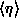and standard deviations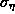of the rms errors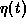given by eq. (7). These values have been extracted from trajectories of 0.4ps duration. In addition, the values for the average energy drifts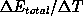as calculated from trajectories of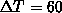ps duration, are given.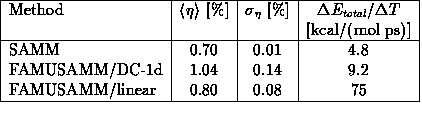Table: Approximation errors of force calculation for various methods as measured by the time averageof the rms error, eq. (7), and by its standard deviation; also given are average energy driftsreflecting algorithmic noise.

As can be seen, the average rms errorsof force approximation are of about the same size (1%) for all three methods. Thevalues of the FAMUSAMM algorithms are only slightly larger than that of SAMM. Thus, the multiple-time-step extrapolation procedures do not seem to sizably reduce the quality of force approximation achieved by SAMM. In contrast, as shown in ref. , cutoff methods exhibit errorswhich are larger by at least a factor of ten. Thus, as far as the quality of force approximation is concerned, the FAMUSAMM procedures essentially preserve the advantageous properties of SAMM.

But considering the fluctuationsof the error, a distinct difference between SAMM and the FAMUSAMM schemes becomes apparent. Due to the application of the multiple-time-step extrapolation procedures, for FAMUSAMM the fluctuationsare tenfold larger than for SAMM. Thus the question arises, whether the slightly larger values ofand the drastically increased values offor the FAMUSAMM methods are accompanied by comparable increases of algorithmic noise.

Consideration of the associated values for the energy drifts in Table i reveals a slight increase for FAMUSAMM/DC-1d as compared to SAMM which is in line with the corresponding increase of. In contrast, a dramatically enhanced algorithmic noise is apparent for the FAMUSAMM/linear approach although in that caseis nearly as small as for SAMM. Hence, the superiority of the DC-1d scheme as compared to the linear extrapolation is actually preserved despite the fact, that in FAMUSAMM extrapolations are applied to local Taylor expansion coefficients representing approximated forces instead to explicitly calculated exact forces. Finally note, that thevalues are uncorrelated to the observed algorithmic noise; this finding underlines the validity of the respective arguments presented in refs.  and .Next: Long time observables Up: Results of quality assessment Previous: Results of quality assessment

Helmut Grubmueller
Wed Apr 30 15:40:09 MET DST 1997